# LM2941 1A Low Dropout Adjustable Regulator

We frequently receive requests for voltage regulators for systems for which the common LM317 is not suitable. Although the LM317 can accept input voltages up to 3-40 Volts, and output regulated voltages from 1.2-37 Volts, it can only output a reliable fixed voltage if the input voltage is at least 2-3 Volts higher than the chosen output voltage – e.g. to get a reliable 12.0V output, the input voltage would need to be 15V+. This voltage difference is the dropout, the amount of voltage dropped (dissipated/wasted as heat) in the regulator.

### Low Dropout Fixed Voltage Regulators

Where a fixed voltage regulator is required, something like the LM2940 range can be used. These are low dropout voltage regulators typically available with fixed output voltages of 5, 10, 12, and 15 Volts.

Such a regulator is perfect for use for example where 12V rated LED spotlights are to be powered with a 12V battery. The battery voltage can range from 12.5 to 14+ Volts, and we need a fixed 12.0V to power the LEDs, so although an LM317 would not be reliable, the LM2940 (which will drop no more than 0.5 Volts) would give a fixed 12.0V output across the full voltage range of the battery reliably.

Unfortunatately we cannot use an LM2940 where a non-standard voltage – e.g. 8.50 Volts – is required.

### LM2941 Adjustable Low Dropout Voltage Regulators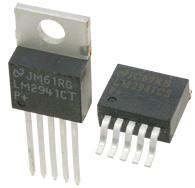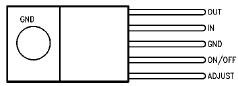LM2941C – Top View

Ideally we want something with the adjustability of the LM317, but the low dropout characteristics of the LM2950. Fortunately such a component exists and it is the LM2941 adjustable low drop out voltage regulator (which is pictured above).

The LM2941 can output voltages from 5.0 to 15.0 Volts * with a typical dropout voltage of just 0.5 Volts (@ 1 Amp, less for lower currents), and an absolute maximum dropout of 1.0 Volts across the full temperature range. The LM2941 can supply up to 1 Amp of current.
* Note that the maximum input voltage is 26 Volts, so this component is not suitable for 24 Volt rated systems.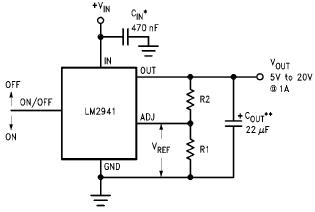Pictured above is the typical application circuit using the LM2941 as an adjustable voltage regulator. The reference voltage (measured across ADJ and GND) is 1.275V. With the resistor R1 typically set to1K, the value of R2 for any given desired output voltage is calculated using the following equation:

R2 = R1 (( Vout / Vref) – 1 ))

For example, if an 8.00V low voltage drop out regulator is required, R1 = 1K, Vref = 1.275, and so R2 = 1000((8.00/1.275) – 1) = 5276 Ohms.

Turning the equation around we can also calculate the output voltage for any given values of R1 and R2:

Vout = Vref * ((R1 + R2) / R1)

…so if we keep R1 as 1K and set R1 as 5K1 (since the 5276 Ohm value calculated is obviously not astandard resistor) we see that Vout = 1.275 ((1000 + 5100) / 1000) = 7.78 Volts. (Note that it is possible to connect standard resistors in series adding up their values to get an exact value if a very accurate voltage regulator is required – e.g. 4K7 + 560R + 10R + 5R6 = 5276 Ohms for an 8.00V regulator)

Click here to view the LM2941 Datasheet (PDF) with sample circuits, and detailed specification data.

### Making a User-Adjustable Voltage Regulator Circuit

Sometimes it is desirable to make a voltage regulator which can have its output voltage adjusted after it has been put together – e.g. if the device to be powered has to be changed for a unit with a different specification. In order to avoid having to put together an all new voltage regulator, the resistor R2 can instead be replaced with a variable resistor (aka potentiometer) such as the one pictured below.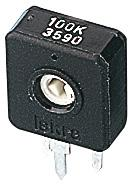The LM2941 can be used reliably for output voltages from 5 to 15 Volts. Setting Vout as 5V and then as 15V, we can calculate that (if R1 is kept at 1K) R2 must be set as 2,900 Ohms for a 5V output, and 10,700 Ohms for a 15V output. Therefore, if R2 is replaced with a 3K resistor in series with a 10K potentiometer, the user can easily change the output voltage through the full range available (since R2 can be set to any value between 3K and 13K).

### Buy LM2941 Voltage Regulator ICs

The LM2941 is available in TO-263 and TO-220-4 packages from Rapid. Click here now to view and buy these LM2941 Adjustable Low Dropout Voltage Regulators.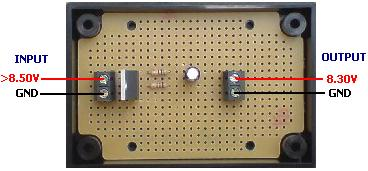Pictured above is an 8.3 Volt low dropout voltage regulator built using an LM2941. With R1 set at 1K and R2 set as 5K6, the calculation suggested that the output voltage would be 8.4V, but the resistors used are only accurate to +/- 5% leading to the difference. Had we required an exact 8.40V regulator we would have selected higher specification resistors, or measured a selection standard resistors to find those that best matched our requirements.

With a load of 200mA the output voltage of this circuit was stable at 8.30V as long as the input voltage was above 8.45V. When the input voltage fell below 8.45V, the output voltage was equal to input voltage minus 0.15V.

If you need a similar low voltage dropout regulator circuit (or an adjustable low dropout voltage regulator) then please email neil@reuk.co.uk with details of your exact requirements.